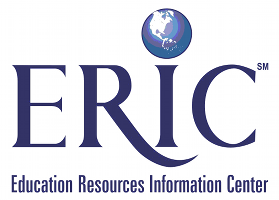You are here:

# Fibonacci Numbers Revisited: Technology-Motivated Inquiry into a Two-Parametric Difference EquationARTICLE

## Sergei Abramovich, Gennady A. Leonov

IJMEST Volume 39, Number 6, ISSN 0020-739X

## Abstract

This article demonstrates how within an educational context, supported by the notion of hidden mathematics curriculum and enhanced by the use of technology, new mathematical knowledge can be discovered. More specifically, proceeding from the well-known representation of Fibonacci numbers through a second-order difference equation, this article explores its two-parametric generalization using computer algebra software and a spreadsheet. Combined with the use of calculus, matrix theory and continued fractions, this technology-motivated approach allows for the comprehensive investigation of the qualitative behaviour of the orbits produced by the so generalized difference equation. In particular, loci in the plane of parameters where different types of behaviour of the cycles of arbitrary integer period formed by generalized Golden Ratios realize have been constructed. Unexpected connections among the analytical properties of the loci, Fibonacci numbers and binomial coefficients have been revealed. Pedagogical, mathematical and epistemological issues associated with the proposed approach to the teaching of mathematics are discussed. (Contains 10 figures and 3 notes.)

## Citation

Abramovich, S. & Leonov, G.A. (2008). Fibonacci Numbers Revisited: Technology-Motivated Inquiry into a Two-Parametric Difference Equation. International Journal of Mathematical Education in Science and Technology, 39(6), 749-766. Retrieved May 24, 2019 from .This record was imported from ERIC on April 19, 2013. [Original Record]

ERIC is sponsored by the Institute of Education Sciences (IES) of the U.S. Department of Education.

Copyright for this record is held by the content creator. For more details see ERIC's copyright policy.